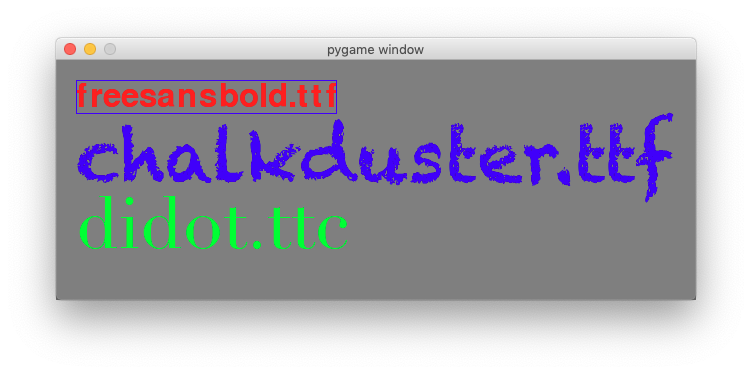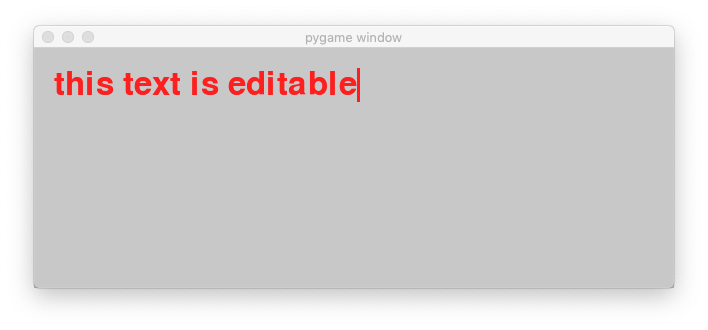# Work with text¶

In pygame, text cannot be written directly to the screen. The first step is to create a Font object with a given font size. The second step is to render the text into an image with a given color. The third step is to blit the image to the screen. These are the steps:

font = pygame.font.SysFont(None, 24)
img = font.render('hello', True, BLUE)
screen.blit(img, (20, 20))


Once the font is created, its size cannot be changed. A Font object is used to create a Surface object from a string. Pygame does not provide a direct way to write text onto a Surface object. The method render() must be used to create a Surface object from the text, which then can be blit to the screen. The method render() can only render single lines. A newline character is not rendered.

## Initialize a font¶

Initializing the font can take a few seconds. On a MacBook Air the the creation of a system Font object:

t0 = time.time()
font = pygame.font.SysFont(None, 48)
print('time needed for Font creation :', time.time()-t0)


took more then 8 seconds:

time needed for Font creation : 8.230187892913818


The function get_fonts() returns a list of all installed fonts. The following code checks what fonts are on your system and how many, and prints them to the console:

fonts = pygame.font.get_fonts()
print(len(fonts))
for f in fonts:
print(f)


You will get something like this:

344
bigcaslonttf
silomttf
sfnsdisplayblackitalicotf
chalkdusterttf
...


## Render the text¶

The font object can render a given text into an image. In the example below, we place a blue bounding rectangle around that text image:

img = font.render(sysfont, True, RED)
rect = img.get_rect()
pygame.draw.rect(img, BLUE, rect, 1)


We then create two more fonts, Chalkduster and Didot at a size of 72 points. We render a text with both fonts:

font1 = pygame.font.SysFont('chalkduster.ttf', 72)
img1 = font1.render('chalkduster.ttf', True, BLUE)

font2 = pygame.font.SysFont('didot.ttc', 72)
img2 = font2.render('didot.ttc', True, GREEN)


Finally the text images are blit to the screen like regular images:

screen.fill(background)
screen.blit(img, (20, 20))
screen.blit(img1, (20, 50))
screen.blit(img2, (20, 120))
pygame.display.update()


This is the result:Here is the full code.

"""Draw text to the screen."""
import pygame
from pygame.locals import *
import time

BLACK = (0, 0, 0)
RED = (255, 0, 0)
GREEN = (0, 255, 0)
BLUE = (0, 0, 255)
GRAY = (200, 200, 200)

pygame.init()
screen = pygame.display.set_mode((640, 240))

sysfont = pygame.font.get_default_font()
print('system font :', sysfont)

t0 = time.time()
font = pygame.font.SysFont(None, 48)
print('time needed for Font creation :', time.time()-t0)

img = font.render(sysfont, True, RED)
rect = img.get_rect()
pygame.draw.rect(img, BLUE, rect, 1)

font1 = pygame.font.SysFont('chalkduster.ttf', 72)
img1 = font1.render('chalkduster.ttf', True, BLUE)

font2 = pygame.font.SysFont('didot.ttc', 72)
img2 = font2.render('didot.ttc', True, GREEN)

fonts = pygame.font.get_fonts()
print(len(fonts))
for i in range(7):
print(fonts[i])

running = True
background = GRAY
while running:
for event in pygame.event.get():
if event.type == QUIT:
running = False

screen.fill(background)
screen.blit(img, (20, 20))
screen.blit(img1, (20, 50))
screen.blit(img2, (20, 120))
pygame.display.update()

pygame.quit()


## Edit text with the keybord¶

The keyboard event can be used to edit a text. First we create a text which we save in a string variable text and which we render to an image:

text = 'this text is editable'
font = pygame.font.SysFont(None, 48)
img = font.render(text, True, RED)


Then we define the bounding rectangle and furthermore a cursor rectangle which is juxtaposed to the text bounding rectangle:

rect = img.get_rect()
rect.topleft = (20, 20)
cursor = Rect(rect.topright, (3, rect.height))


Inside the event loop we watch out for KEYDOWN events. If the key press is a BACKSPACE and the lenght of the string is larger than 0, then we remove the last character, else we append the new character to the text variable:

if event.type == KEYDOWN:
if event.key == K_BACKSPACE:
if len(text)>0:
text = text[:-1]
else:
text += event.unicode


Then we render the modified text, update the bounding rectangle, and place the cursor box at the end of the updated bounding rectangle:

img = font.render(text, True, RED)
rect.size=img.get_size()
cursor.topleft = rect.topright


In order to make the cursor more visible, we let it blink every 0.5 seconds. We do this using the time.time() floating point value:

screen.fill(background)
screen.blit(img, rect)
if time.time() % 1 > 0.5:
pygame.draw.rect(screen, RED, cursor)
pygame.display.update()


This is the result:Here is the full code.

"""Edit text with the keyboard."""
import pygame
from pygame.locals import *
import time

BLACK = (0, 0, 0)
RED = (255, 0, 0)
GRAY = (200, 200, 200)

pygame.init()
screen = pygame.display.set_mode((640, 240))

text = 'this text is editable'
font = pygame.font.SysFont(None, 48)
img = font.render(text, True, RED)

rect = img.get_rect()
rect.topleft = (20, 20)
cursor = Rect(rect.topright, (3, rect.height))

running = True
background = GRAY

while running:
for event in pygame.event.get():
if event.type == QUIT:
running = False

if event.type == KEYDOWN:
if event.key == K_BACKSPACE:
if len(text)>0:
text = text[:-1]
else:
text += event.unicode
img = font.render(text, True, RED)
rect.size=img.get_size()
cursor.topleft = rect.topright

screen.fill(background)
screen.blit(img, rect)
if time.time() % 1 > 0.5:
pygame.draw.rect(screen, RED, cursor)
pygame.display.update()

pygame.quit()